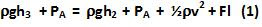## Sunday, July 19, 2009

### Irodov Problem 1.335First let us understand what is happening in the problem. As the liquid moves along the tube it has to work against the viscous forces and so it looses energy. From Bernoulli's equation we know that the energy per unit volume is given by. v is constant throughout the tube since the rate volume of water flowing should be the same at all points in the tube. h is constant since the tube is horizontal. This means, the pressure head P, is changing due to the work done by the viscous forces. Thus, as water flows along the tube, the pressure head reduces and this is reflected by reduction in height of the water columns h2 and h1.

Let the viscous force acting on the fluid be F. This means that the work done by the viscous forces as the liquid moves a distance of x is given by Fx and this will be also equal the loss in energy of the fluid. Hence, Bernoulli's equation will have an Fx term in addition as well to account for the loss due to work done against viscous forces.

Let us consider the energy per unit volume at points A and B. The fluid at point A is still i.e. has zero velocity and the pressure is. The pressure at point B iswhile the fluid moves with a velocity v. Also the fluid has lost an energy of Fl working against the viscous forces. Hence we have,Similarly considering points A and D we have,From (1) and (2) we can write,Now let us consider points A and E. At E, the fluid enters the atmosphere and so we have,